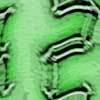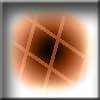#### You may also like### Times

Which times on a digital clock have a line of symmetry? Which look the same upside-down? You might like to try this investigation and find out!### Penta Play

A shape and space game for 2, 3 or 4 players. Be the last person to be able to place a pentomino piece on the playing board.### Clocks

These clocks have been reflected in a mirror. What times do they say?

# Transformation Tease

##### Age 7 to 11Challenge Level

Amy, David, Euan, Lewis and Robert at St. Nicolas School, Newbury tried solving this problem. They have explained their solution very clearly although it is quite long!

The shape ABCD is a trapezium. We think the coordinates are A (4,2) B (6,2) C(7,1) D (3,1)

After moving 3 squares left and 4 up the new coordinates are A (1,6) B (3,6) C (4,5) D (0,5). We noticed that the x coordinate of the new number was 3 less than the original coordinate and the y coordinate was 4 more than the original coordinate.

We reflected the shape in the x axis. The new coordinates are A (4,-2) B(6,-2) C (7,-1) D (3,-1). The x coordinate stayed the same but the y coordinate has got a minus in front of it. We predicted the new coordinates after reflecting in the y axis A (-4,2) B (-6,2) C (-7,1) D (-3,1)

We reflected the original shape in the line y = -x. The new coordinates we found were A (-2,-4) B (-2,-6) C (-1,-7) D (-1,-3). These coordinates are the ones we came up with when we predicted reflecting the 3 points in the line y = -x. (-4,-2) (4,-6) (5,5)

When we took the original shape and rotated it anticlockwise about the origin, we came up with these coordinates A (-2,4) B (-2,6) C (-1,7) D (-1,3)

Looking at the patterns we found, this transformation could also be described as reflecting in the line y = -x and then reflecting in the x axis.
Example A starts (4,2), after reflecting in the line y = -x it is (-2,-4), and then reflecting in the x axis it is (-2,4), which is the same as rotating through 90 degrees.